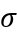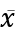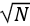# How To Find Standard Error Of The Mean In Excel, SPSS, R

In this lesson, we are going to learn how to find standard error of the mean in Excel, SPSS, and R with step-by-step examples and clear explanations.

We are going to touch briefly on what standard error is, the equation for standard error, and its use in statistical research.

You will be able to download the sample files used in this lesson and follow along. Here are the main learning outcomes you expect to get once completing this lesson:

Learning Outcomes

• Know what the standard error of the mean is as well as the standard error equation.
• Learn how to calculate the standard error of the mean in Excel.
• Learn how to compute standard error in SPSS.
• Learn how to find standard error in R.

Please note that the terms standard error and estimated standard error of the mean are used interchangeably in statistics. However, they point exactly to the same thing.

Without further ado, let’s get started.

## What is the Standard Error of the Mean

In an ideal world, researchers would have superpowers to survey an entire population without the need of dealing with samples. But we know that’s not the case.

In most cases, we collect data by sampling the population we investigate. If we take multiple samples of the same population we will observe that they are slightly different.

For instance, the mean of each sample will be different from another as each sample likely contains different members of the same population.

So how do we know if sample data represent a whole population?

Say hello to the standard error of the mean or simply, standard error. And here are the notations used by various authors in the literature:

The standard error is used to determine how close the mean of a sample taken from a population is compared to the average value of the true population.

A lower standard error value shows that the sample means have a close distribution around the population mean therefore more representative of the true population.

In contrast, a higher standard error value likely shows that the sample is an inaccurate representation of the true population.

The best way to lower a high standard error value is by increasing the sample size. We will do a comparison later in this lesson.

Is also important to use random sampling when collecting the data to avoid sampling bias.

Finally, you should really understand the difference between the standard error of the mean and standard deviation in statistics. The two are often a source of great confusion among students.

## How To Find Standard Error of the Mean

The below formula is the equation for the standard error of the mean using the population standard deviation:

Where:= actual population standard deviation= mean of x scores= square root of the sample size

The caveat here is that we need to know the actual population standard deviation to be able to calculate the standard error using the above formula.

Usually, populations are large, and is unlikely we have access to the entire population to calculate the population standard deviation value.

As with most cases in research, sampling a population is a much easy and less costly approach. And luckily enough, there is a way to estimate the standard error of mean using the sample standard deviation too. Hooray! Here is the formula:

Where:

S = sample estimate of the standard deviation= mean of x scores= square root of the sample size

You may have noticed that both formulas for the standard error above are quite similar. Indeed they are, the only difference being whether we know the value for population standard deviation or not.

Now that we know the equations for standard error, let’s do some basic math and learn how to calculate standard error by hand.

Example 1: Assuming we know that the actual standard deviation of a population consisting of students taking their final exam is 7. We also took a random sample of 100 respondents from this population. Therefore we have:

Since in this standard error example we know the actual standard deviation value, we will use the first equation for the standard error above, respectively:

After plugging the numbers in the equation we have:

So, how do we interpret the standard error result in this example? It simply means that when we take a random sample of N = 100 from this population, the average difference between sample means and the population mean is 0.70

Example 2: Now, let’s assume we don’t know the population standard deviation of the students taking their final exam in our school. Instead, we know the sample standard deviation (S) which let’s assume is 5. The sample (N) remains unchanged, respectively 100. Therefore we have:

This time we will use the equation for standard sample deviation:

After we insert the numbers we have:

We can interpret this result as the average difference between sample means and the population mean when the sample is randomly selected and N = 100 is 0.50.

The standard error of the mean is a measure of how much difference you expect to get between a sample statistic and the population parameter and the sample means and the population mean when samples are randomly selected and of a given size.

## Calculate Standard Error in SPSS

There are numerous ways to find the standard error in SPSS. In this section, we will focus on two methods that require the least amount of clicks :).

If you wish to practice along, download the SPSS dataset used in the following standard error example HERE.

Launch SPSS on your computer, navigate to File → Open →  Data, select the file with the .sav extension you download above and press the Open button to import it in SPSS.

(1) Calculate Standard Error of the Mean in SPSS using Explore Analysis

In SPSS top menu, navigate at Analyze → Descriptive Statistics → Explore

On the Explore window, select a variable in the left box and click the “arrow” button to add it to the Dependent List.

Click the OK button to proceed with the analysis.

On the Output window, scroll down to the Descriptive table. On the Mean row, check the Std. Error column to find the standard error value for the respective variable.

In our example, the mean for the System variable is 4.10 and the standard error is 0.081.

This standard error value is a fairly low one meaning that the sample means analyzed are closely distributed around the population mean. In other words, our sample is representative of the population from where was taken.

If the standard error of the mean in your analysis is high, increasing the sample size through random selection will likely decrease the standard error value.

For instance, if your sample size is N = 50, you could increase it to N = 100 or more to lower the standard error, as seen in the side-by-side comparison below.

Pay attention to the mean and standard error of the mean in both figures.

(2) Find Standard Error on the Mean in SPSS using Frequency Analysis

Another way of calculating standard error in SPSS is using the Frequency analysis. In SPSS, navigate to Analysis → Descriptive Statistics → Frequencies

On the Frequencies window, add the variable of interest from the left box to the Variable(s). Uncheck the Display frequency table check-box and click the Statistics button.

On the Statistics window, make sure the S.E. mean check-box is selected, and press the Continue button.

Click on the OK in the Frequencies window to proceed with the standard error analysis.

The standard error value will be shown in the Std. Error of Mean row of the Statistics table

## Standard Error Calculation in Excel

To find the standard error of the mean in Excel, all we need to do is to translate the equation for standard error respectively standard error = standard deviation / square root of the total number of samples N to Excel formula. First, download the Excel dataset used in this example HERE.

1. On your Excel data set, click on an empty cell anywhere on your datasheet

2. Copy the standard error Excel formula below into the Insert Function field in Excel.

=STDEV(sampling range)/SQRT(COUNT(sampling range))

3. Replace the “sampling range” in the standard error Excel formula with the actual range of cells you want to include in your analysis.

Once your selection is done, hit the ENTER key to complete the analysis.

Excel will show the standard error result in the respective cell as seen in the capture below.

## Standard Error Calculation In R

Finally, let’s have a look at which function we can use to find the standard error of the mean in R.

As we discussed earlier, the standard error of the mean is just the standard deviation divided by the square root of the sample size.

If you want to practice the standard error in R analysis along, download the Excel dataset used in this guide HERE.

Launch RStudio on your computer. On the R top menu, navigate to File → Import Dataset → From Excel.

On the Import Excel Data in R window, click on Browse and select the dataset.xlsx file you download above. Click Open than the Import button to finish importing the Excel dataset in R.

NOTE: R might require certain libraries to be able to import data from Excel files (.xlsx, .csv, etc.). If prompted, allow RStudio to automatically install the required dependencies.

(1) Find Standard Error in R using the Standard Error Formula

The first step is to translate the equation for standard error of the mean to a new function(x) in R. Let’s call this function, e.g., stderr or any other name you want.

Type this function in the Console window in R then press ENTER.

stderr <- function(x) sd(x)/sqrt(length(x))

Next, let’s calculate the standard error of the mean for the age column in our dataset.

To do so, we will basically invoke the function we created and specify the dataset file and column we want to compute the standard deviation in R using the following syntax:

stderr(dataset$age) Where: stderr = function for standard deviation formula in R dataset = the dataset file we imported in R age = the column (variable) we want to find the standard deviation of the mean value. The standard deviation for the age variable in our dataset is 0.06 as seen in the capture below: (2) Find Standard Error in R using Plotrix library This method uses the std.error() function in the Plotrix package in R. First we need to install the Plotrix library by typing the following command in the Console window in R: install.package('plotrix') Next, we need to call the Plotrix library in R using the following command: library('plotrix') Finally, we can use the std.error function to calculate the standard error of the mean for the system variable in our dataset. std.error(dataset$system)

## Wrapping Up

Standard error of the mean measures the difference between the sample means compared with the mean of the real population. In other words, it tells us if our sample is representative or not for the population from where was taken.

If the sample analysis shows a high standard error, the best way to lower it is by increasing the sample size using random data collection.

Finally, if you have any suggestions to improve this post further or if you need help with your research paper, don’t hesitate to contact me.

Uedufy (November 30, 2023) How To Find Standard Error Of The Mean In Excel, SPSS, R. Retrieved from https://uedufy.com/how-to-find-standard-error-of-the-mean-in-excel-spss-r/.
"How To Find Standard Error Of The Mean In Excel, SPSS, R." Uedufy - November 30, 2023, https://uedufy.com/how-to-find-standard-error-of-the-mean-in-excel-spss-r/
Uedufy March 15, 2022 How To Find Standard Error Of The Mean In Excel, SPSS, R., viewed November 30, 2023,<https://uedufy.com/how-to-find-standard-error-of-the-mean-in-excel-spss-r/>
Uedufy - How To Find Standard Error Of The Mean In Excel, SPSS, R. [Internet]. [Accessed November 30, 2023]. Available from: https://uedufy.com/how-to-find-standard-error-of-the-mean-in-excel-spss-r/
"How To Find Standard Error Of The Mean In Excel, SPSS, R." Uedufy - Accessed November 30, 2023. https://uedufy.com/how-to-find-standard-error-of-the-mean-in-excel-spss-r/
"How To Find Standard Error Of The Mean In Excel, SPSS, R." Uedufy [Online]. Available: https://uedufy.com/how-to-find-standard-error-of-the-mean-in-excel-spss-r/. [Accessed: November 30, 2023]

## References

Field, A., Miles, J., & Field, Z. (2012). Discovering statistics using R. SAGE Publications.

Field, A. (2013). Discovering statistics using IBM SPSS statistics (4th ed.). SAGE Publications.

McNeil, E. (2020). Data management and visualization using R.– Songkhla: Epidemiology Unit, Faculty of Medicine, Prince of Songkla University, 2020.##### Leonard

Leonard is a Ph.D. student in Data Science and holds an MBA and B.Sc. He has an impressive public speaking profile on education, engineering, and research. He loves to help students achieve their academic objectives and believes education is the key to building a better future for mankind.

Articles: 51